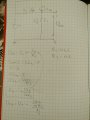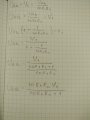# How to change electric scheme with Tevenen generator

Thread Starter

#### awesommee

Joined Nov 18, 2019
13
First of all I am from Serbia and I don't know English terminology well. This is my homework http://tnt.etf.bg.ac.rs/~si1oe/Domaci zadaci/Prvi_domaci_zadatak_2019_2020.pdf
Ignore all the text or translate it on google translate(but it is not necessary).Ignore part in blue box right of A and B points as it doesn't exist. I need to change scheme left of points A and B with Tevenen generator. I found equivalent R for it and it is 500Ω. Now I need to find voltage(U) between points A and B. I am not sure how to do it. I think it is 0V, but I am not sure. Can you tell me how do I find that out? Once again sorry for bad English terminology.

#### Wolframore

Joined Jan 21, 2019
2,281
There is voltage between A and B. Think through it using KVL

Thread Starter

#### awesommee

Joined Nov 18, 2019
13
There is voltage between A and B. Think through it using KVL
What is KVL?

#### Wolframore

Joined Jan 21, 2019
2,281

#### Jony130

Joined Feb 17, 2009
5,230
It seems to me that TS whats to find a Thevenin equivalent circuit for a left side of a circuit.

I found equivalent R for it and it is 500Ω.
I've got the same resolut.

I think it is 0V, but I am not sure.
Yes, but only if for V1 = 0V. What if V1 is equal to 10V? What is the Vth voltage?
Additional I suspect that you need to find Vth in terms of a V1 voltage.

Thread Starter

#### awesommee

Joined Nov 18, 2019
13
It seems to me that TS whats to find a Thevenin equivalent circuit for a left side of a circuit.

Yes, but only if for V1 = 0V. What if V1 is equal to 10V? What is the Vth voltage?
Additional I suspect that you need to find Vth in terms of a V1 voltage.
You are totally right for both statements. Here is how I tried to find voltage between A and B for any V1 value and it doesn't seem right when I test it in pc simulation.Can you point me out where I made a mistake?

#### Jony130

Joined Feb 17, 2009
5,230
where I made a mistake?
You forgot the Ohm's law V = I * R therefore
Vab = V1 - Ib*R1

Next time track your units properly.

Thread Starter

#### awesommee

Joined Nov 18, 2019
13

#### Jony130

Joined Feb 17, 2009
5,230
But all this math is not needed. This circuit with CCCS (Current controlled current source) and R1 resistor form a "resistance converter" (we have a similar situation in BJT's Emitter follower). The equivalent resistance seen by R2 is Rth = R1/(β + 1). And now we can use a voltage divider equation:

Vab = V1 * R2/( R1/(β +1) + R2 ) = V1/2

Or R2 resistance is seen by V1 as a (β + 1)*R2 resistance.